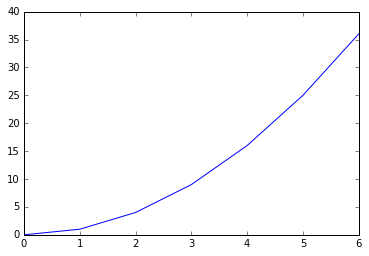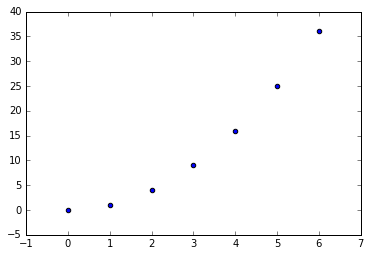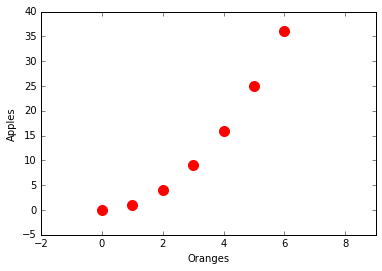In :
# This line is only needed if you're using a jupyter notebook
# if you don't know what that is, you're probably not using one :)
# don't include it if you're only writing a script
%matplotlib inline

# This imports all the libraries we need
import matplotlib
import pylab as pl
import numpy as np


Let's create a file called mydata.txt

In :
filename = "mydata.txt"
f = open(filename, "w")


Let's create two lists of numbers we'll write to it: x and y

In :
x = [0, 1, 2, 3, 4, 5, 6]
y = [0, 1, 4, 9, 16, 25, 36]


writing to the file

In :
# for every value in x and y we need to write a line
# python needs us to convert the number to a string first (str), and then add a newline character at the end
for (xvalue, yvalue) in zip(x,y):
f.write(str(xvalue) + " " + str(yvalue) + "\n")

# close the file after writing
f.close()


Now, let's open it

In :
data = np.genfromtxt("mydata.txt")
print(data)

[[  0.   0.]
[  1.   1.]
[  2.   4.]
[  3.   9.]
[  4.  16.]
[  5.  25.]
[  6.  36.]]


Let's assign names to the first and second columns

In :
xx = data[:,0]
yy = data[:,1]

In :
print(xx)

[ 0.  1.  2.  3.  4.  5.  6.]

In :
print(yy)

[  0.   1.   4.   9.  16.  25.  36.]


Now, let's plot it

In :
pl.plot(xx,yy)

Out:
[<matplotlib.lines.Line2D at 0x1105b8ef0>]You can also plot it as a scatter plot

In :
pl.scatter(xx,yy)

Out:
<matplotlib.collections.PathCollection at 0x1106b4da0>Let's create a figure, so that we can control how it's plotted

In :
fig = pl.figure() # Create figure

ax.set_xlabel("Oranges")
ax.set_ylabel("Apples")

# Tweak the color and size of the scatter points

ax.scatter(xx, yy, color='red', s=100)

# change the axes limits
ax.set_xlim(-2,9)

Out:
(-2, 9)Save the plot as an image

In :
fig.savefig("mydata.png")